TECHNISCHE INFORMATIONEN

Technical İnformation On The Pipe Bend

1. Definition of Tube Bending

Tube bending is a process used to reshape tubing or piping into a certain shape or design. This process works on metal, alloy, aluminum, titanium, and steel.

Various methods are used for bending tubes depending on the material in use and the required precision. The more common procedures are rotary draw bending, 3-roll bending, compression bending and ram bending (press bending) (Figure 1).Figure 1. Bending processes.

2.1. Process Types

2.1.1. Rotary Draw Bending

When rotary draw bending is applied, the tube is fastened between the bend die and the clamp die. The rotation of both tools around the bending axis bends the tube to the radius of the bend die. The pressure die (slide piece) serves the purpose of receiving the radial stress, which is generated during the forming process, and supports the straight tube end from outside. If a mandrel and wiper die are additionally applied (mandrel bending), a high quality work piece can be achieved even with thin-walled pipes and tight bending radius.

2.1.2. Compression Bending

Compression bending is similar to rotary draw bending. This bending method is carried out with a sliding carriage and a stationary bend die, between which the tube is clamped. The sliding carriage, which rotates around the radius block, bends the tube to the radius of the bend die.

2.1.3. Ram Bending (Press Bending)

When ram bending is applied, bending tool is pressed against two counter-rollers, either manually or by means of hydraulics. This motion forces the tube inserted between the radius block and the counter-rollers to bend around the radius. The tube cannot be supported from within; therefore this method is suitable for thick-walled tubes and large bending radius only.

2.1.4. 3-roll Bending

3-roll bending is also used for producing work pieces with large bending radii. The method is similar to the ram bending method, but the working cylinder and the two stationary counter-rollers rotate, thus forming the bend .

3. Bending Tools

Bending tools for Rotary Draw Bending (Figure 2) were designed during this project. Bend die is the main tool which directs the tube along the rotation axis. Clamp die meets the bend die and moves simultaneously as it is used for clamping the workpiece. Mandrel is used for preventing the bending area from section shape changes and collapse.Figure 2. Bending Tools .

Pressure die is designed to compensate the bending forces and for keeping the tube in the right position it moves linearly along with the tube. Wiper die is fixtured between the cavity of the bend die and the tube immediately behind the line of tangency. The critical feature of a wiper is the feathered edge, which prevents the terminal hump from exceeding its yield point and setting into a wrinkle . Due to small radius of the tube and sufficient wall thickness, wrinkling will not be crucial so wiper die is not used in order to reduce cost.

Detailed explanations of these tools will be given in Tool ion section.

4. Applications

Tube bending has a wide range of applications. Bended tubes and pipes are used for business signages, structural purposes, agriculture (e.g. tractor), automobile parts, ornamental and furniture parts (Figure 3).

Connection points and standard dimensions of the tools such as die height are designed according to this machine.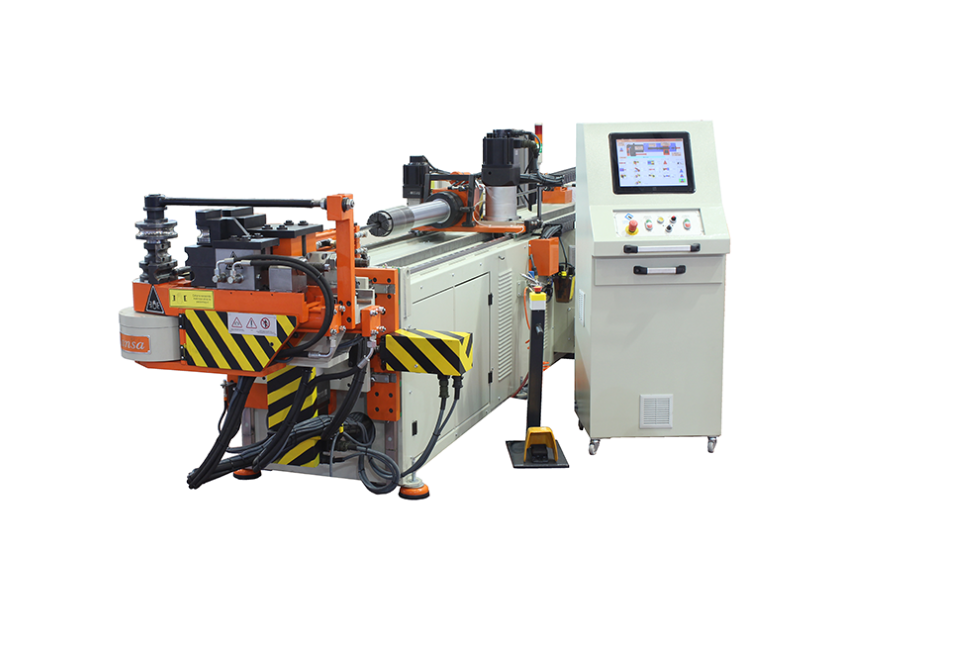Figure 4. Bending machine.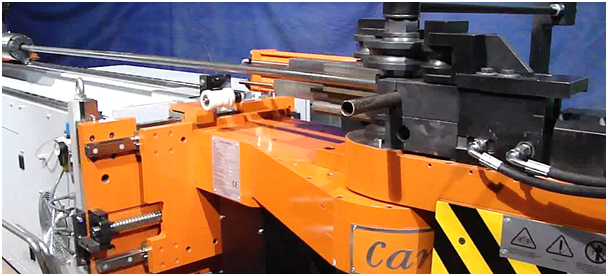Figure 5. Tube bending process.

The brass tubes to be bend are shown in Figure 6. and 7.. They have different geometries but the bended parts have the same dimensions, therefore the designed die will be used for both of the tube.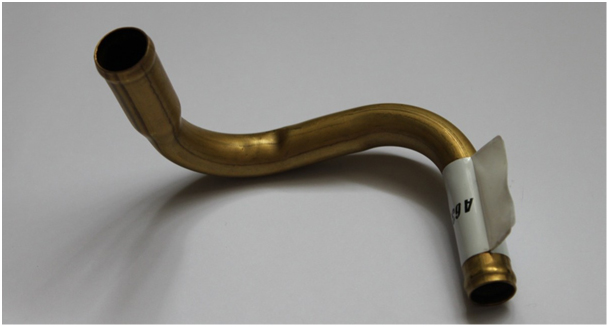Figure 6. - 7. Tubes.

2. Problems in Tube Bending

2.1. Important Features

Appearance: It is considered important where aesthetical appearance becomes one of the major concerns where the tube itself is mounted on an area visible to the human eye, or simply where the tube itself is a part of an ornamental structure. Flattening should be prevented in order to maintain good appearance.

Wall thickness: In some applications, where any pressured fluid passes inside the tube, wall thickness is reckoned to be important, since thinner the walls, smaller the force that it can withstand without being damaged.

Geometry: Different tube geometries may require different parameters when forming. For instance, substantial care must taken when forming square tube profiles, Since it is much more difficult to sustain the sharp edges of the profile in the bending processes.

2.2. Factors Causing Difficulties

Low wall thickness compared to tube diameter: When the ratio of wall thickness to tube diameter is small to a considerable amount, fracture and wrinkling may occur on the tube walls. There is a limit to the ratio in question for every ed tube material.

Low bending radius: Change in the bend die diameter changes the bending radius. Bending with low bending radiuses may be critical, since there will be too much tension on the outer side and too much compression on the inner side of the tube which can cause tearing or collapse from the outer side and wrinkling on the inner sides. Figure 8. shows a tube after bending process with a small diameter bend die. If the diameter of the bend die was bigger, the tube would be bended without flattening.Figure 8. Bending with small diameter bend die.

Weld: Care must be taken when bending tubes preformed with welding. The bending should not be made through the welded area since the welded face may not withstand the tensile forces and may result in failure through its weld seam.

2.3. Bending Problems

Section changes: Due to the tensile and compressive stresses in the outer and inner sections respectively, outer thinning and inner thickening occur on the cross-section of the tube in the bending area.(Figure 9)Figure 9. Section changes .

Wrinkling: When working with low bending radius and low wall thickness compared to tube diameter conditions, wrinkling can occur (Figure 10). To prevent this, proper wiper element should be used.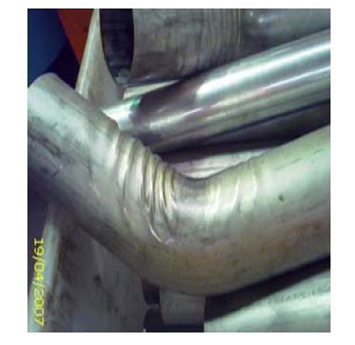Figure 10. Wrinkling .

Hump on outside of pull off end: There is a clearance of 1mm between bend die and clamp die when holding the tube to bend. This clearance is used so as to better hold the part to bend and prevent it from sliding. Nevertheless, if the bend die and clamp die are to hold the part too tight, humps can be generated through the clearance and it is undesirable.

Scratches in clamp area: Scratches in the clamp area can occur if the clamp hold the part too tight.

Ball segment bumps in bend area: If the mandrel used had balls and if the size and material of the mandrel was not ed properly, there may be ball prints in the bend area that can be seen from the outside.

Scratches on inside of bend area: Mandrel used can make scratches on the inside of the bended area of the tube. Therefore, to overcome that problem, appropriate mandrel radius and material must be ed.

Tooling marks on centerline: Pressure die serves the purpose of receiving the radial stress and supports the straight tube from outside. While doing this, it should permit the tube to slip inside it. Because of this, if the pressure die material is not ed properly, tooling marks on centerline can occur. For instance, ductile cast iron can be used as the material which has spherical grains in the microstructure range. That way, tooling marks can be prevented.

Springback: When the bending process will be over, the remained stresses in the body of the bended tube will cause to a relaxation in the part that will decrease the degree of bending (Figure 11). To solve the problem, the initial degree of bending might be increased to a certain degree driven by the simulation results. Another solution relies on increasing the elastic modulus (E) of the tube material. Increasing the E means making the part more rigid which will result less loosening of the part after the forces are removed.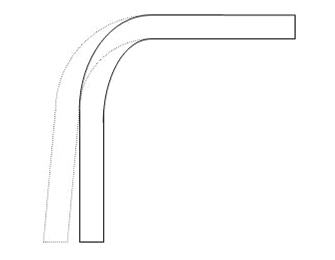FFigure 11. Springback .

Collapse: If the proper mandrel is not used when bending tubes of low wall thickness to diameter ratio, the outer part of the tube to be bent may not handle the tensile forces and may collapse. Proper mandrel with proper ball size should be ed. Also bending radius must be big enough to prevent collapse (Figure 8).

Tearing: Tearing may occur in parts with small bending radius. Bended part cannot withstand the tensile forces generated on the outer side of the tube and can tear out (Figure 12). For this, proper material ion should be made.Figure 12. Tearing .

3. Tube Design

3.1. Tube Material ion

Mercedes Benz Türk gave the project, so tube material and geometry was already determined. Since it is not acceptable just to except it, material comparison is done for radiator tubes between Aluminum, Copper-Brass and Stain less Steel.

Radiator tube material needs to have high thermal conductivity, high melting point, high tensile strength and high corrosion resistance in order to prevent failure from hot, highly pressured liquid passing through the tubes. It also needs low specific weight for lighter structures and good solderability for soldering the fins to the radiator.

Material ion

Mechanical and physical properties of Aluminum AA 3003, Brass UNS C26000 and Stainless Steel are shown in Table 1.

UNS represents Unified Numbering System of United States. UNS C26000 is a Cartridge Brass, 70% grade Wrought Copper Alloy. It is commonly called UNS C26000 Copper-zinc brass. It is composed of (in weight percentage) 70.0% Copper (Cu) and 30.0% Zinc (Zn). 

AA represents Aluminum Association. AA3003 is called Alclad wrought Aluminum alloy composed of %98.6 Aluminum (Al) with %0.12 Cu and %1.2 Manganese (Mn) , .

 . Material Density (g/cm^3) Thermal Conductivity (W/m°C) Tensile Yield Strength (MPa) Modulus of Elasticity (GPa) Coefficient of Thermal Expansion ( μm/m°C) Melting Point (°C) Brazing Temperature (°C) Aluminum AA 3003 2,75 160,0 145 70 23,2 643-655 ~600 Brass UNS C26000 8,53 120,0 435 110 19,9 915-955 ~600 Stainless Steel 7,75 16,3 515-827 190-210 17,3 1371-1454 620-1150

Table 1. Tube material comparison.

Stainless steel could be used due to its high tensile strength and high corrosion resistance, but it is very expensive and has a very low thermal conductivity that makes it redundant for the usage area.

Aluminum has lower density and better conductivity than brass, but radiator tube strength is equally, if not more, important. Brass, of course, is considerably stronger than aluminum. This overall strength allows for thinner walled tubes to compensate for the difference in conductivity and weight.

Taking advantage of these characteristics – superior thermal conductivity, strength and corrosion resistance – manufacturers can use thinner material throughout and thereby design a

copper/brass radiator having a lower total weight than one made from aluminum, but with the same or better heat rejection capacity. 

Competitive advantages of Copper/Brass versus Aluminum are shown in Table 2:

 Technical Commercial High thermal conductivity Lower fabrication cost High corrosion resistance Well-established recycling tradition (infrastructure in place) High tensile yield strength High scrap value Higher melting point Well-established aftermarket tradition (infrastructure in place) Lower coefficient of thermal expansion High-quality reputation Higher modulus of elasticity Easy repairability

Table 2. Advantages of Brass over Aluminum.

3.2. Plastic Deformation Analysis in Tube Bending

As indicated by N. C. Tang , in tube bending, stresses separated into three components which are longitudinal σx, circumferential σc and radial stresses σr. Since the wall thickness is much smaller than the radius of the tube, radial stress can be neglected. Also the formulas are changing according to the inner and outer sections of the tube. As shown in Figure 13, for the values from 0° to 90° of α is the outer semi-circle of the tube and from 90° to 180° of α is the inner semi-circle of the tube.Figure 13. Outer and inner semi-circles of the tube .

The geometry parameter of the tube is defined as: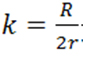(1)

(1)

Where R is the desired bending radius of the tube and r is the mean radius of the tube cross-section shown in Figure 14.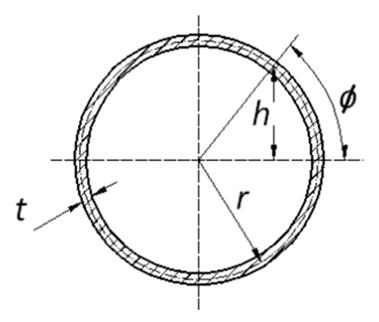Figure 14. Cross-section of the tube .

3.2.1. The stress in the outer semi-circle

In the outer semi-circle, the longitudinal stress σx is tensile, and the circumferential stress σc is compressive stress. From the maximum-shear-stress theory, the relation between the two stresses is:Where σs is the yield strength of the tube material. Also longitudinal and circumferential stresses can be expressed as: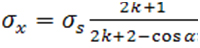(2)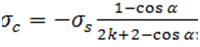(3)

3.2.2. The stress in the inner semi-circle

In the inner semi-circle, both σx and σc are compressive stresses. From the maximum-shear-stress theory, the relation between the two stresses is: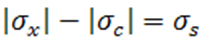(4)

Also longitudinal and circumferential stresses can be expressed as:(5)(6)Figure 15. Stresses for inner and outer sections.

Figure 15 is a Matlab graph which shows the results of the stress formulas indicated so far. Vertical axis shows the angle defined in Figure 13 and horizontal axis shows stress values. Longitudinal σx and circumferential σc stresses are indicated in blue and green respectively. As seen from the graph, in the outer section the longitudinal stress is positive which means it is a tensile stress. And for all other values, the stresses are compressive.

3.2.3. Outer thinning

Wall thickness changes by α and for the outer semi-circle the thickness can be defined as:(7)(8)

Where to is the thickness in the outer semi-circle and t is the original thickness of the tube before bending.

The smallest thickness is located at the outermost point. By substituting α = 0 , the smallest thickness can be expressed as: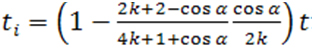(9)

3.2.4. Inner thickening

For the inner semi-circle the thickness can be defined as::

Where ti is the thickness in the inner semi-circle.

The largest thickness is located at the innermost point. By substituting α = 180 , the smallest thickness can be expressed as:(10)Figure 16. Outer thinning and inner thickening of the tube.

Figure 16 is a Matlab graph which shows the outer thinning and inner thickening respectively up and down according to horizontal axis to check whether an anomaly exist or not.

3.2.5. Circumferential shrinking

The shrinking of the diameter is caused by the circumferential stress on the tube cross-section.

Average diameter „ds? and perimeter „C? after bending are expressed:ve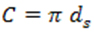where d is the inner diameter of the tube.

3.2.6. Neutral axis deviation

Since longitudinal stress in the outer half is smaller than in the inner half, and the outer wall is thinner than the inner wall for balance of the moment of the internal force, the neutral axis should move to its inner side as shown in Figure 17.

This deviation can be expressed as:(12)Figure 17. Natural axis deviation and flattening .

This deviation can be expressed as3.2.7. Flattening

Flattening critical point can be defined whenKesit oran birimi olan W ve şöyle hesaplanabilir: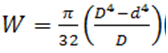Where W is the section modulus which can be calculated as:

Where D is the original outer diameter of the tube.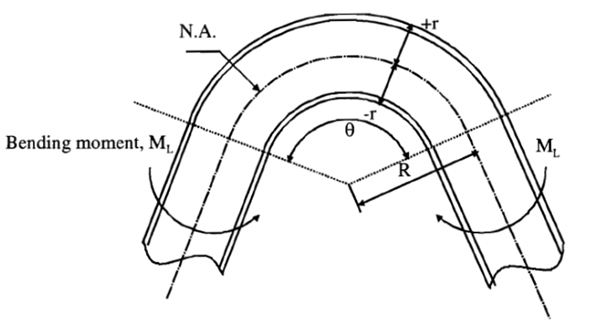Figure 18. Bending Moment .

3.2.9. Feed preparation length

After bending, the geometric centroid axis arc length (R x θ) is larger than the actual neutral axis length. Feed preparation length L can be calculated as:(16)

Where θ is the bending angle.

3.2.10. Springback

As Sözen indicated , while the machine load released from the bent tube, the total bend angle decreases and the radius of bend increases simultaneously due to the springback shown in Figure 19. In analytical calculations, it is assumed that the total bending length in the deformation zone remains the same at all time. Total bending length can be written as:Where θL and RL are the bending angle and bending radius respectively in loaded condition and θU and RU are the parameters for unloaded condition.

Bending angle for unloaded condition can also be written as:(18)

Where E is the young modulus and I is the moment of inertia of the tube which can be calculated as: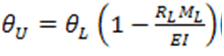(19)

Bending angle for unloaded condition can also be written as: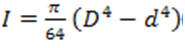(20)

Figure 19. Springback .3.2.11. Analytical calculations

Analytical calculations made by writing a Matlab code and the results are shown in Table 4. In order to determine the values, formulas were used given so far and in Table 3, 6 basic geometrical and mechanical parameters are entered in the code and results are calculated. These calculations are valid just for bending a straight tube that does not have any flange in the head. For this project, plastic deformation analysis, made on Dynaform, are shown for the flanged tube in the further sections.

 Definition Value Unit Outside Diameter 15 mm Wall Thickness 1 mm Bending Radius 35 mm Bending Angle 90 Deg Yield Strength 435 MPa Young Modulus 110 GPa

Table 3. Basic properties of the tube.

 Definition Value Unit Outer Thinning 0.90 mm Inner Thickening 1.16 mm Inner Diameter shrinking 4.85 % Neutral Axis Deviation 1.18 mm Bending Moment 99.13 Nm Unloaded Bend Angle 87.38 Deg Unloaded Bend Radius 36.05 mm Over Bend Angle 92.70 Deg Over Bend Radius 37.13 mm Preparation Length 56.51 mm Flattening Critical Point 168.07 mm

Table 4. Matlab analytical calculation results.

In Table 4, Unloaded Bend Angle and Unloaded Bend Radius shows the results for bending the tube by 90° (Bending Angle – Table 3). That means if the tube is bended by 90°, due to the springback, Unloaded Bend Angle becomes 87.38° and Unloaded Bend Radius becomes 36.05 mm. In order to get the desired bending angle 90°, Over Bend Angle should be 92.7° and Over Bend Radius becomes 37.13 mm as indicated in Table 4.

4. Tool Design

4.1. Tool Material ion

Tool materials are ed according to the information acquired from the company visits. Firstly alternative materials are discussed, and then material ion is made.

Ductile cast iron

Firstly, the pressure die part material is discussed. The pressure die should let the material flow without losing its shape because this part stays stable during the bending operation. Ductile cast iron lets the material flow and due to its spherical grains, the flow results without undesired scratches on the surface of the tube. It is stated that ductile cast iron is the most proper material for the pressure die part because of the properties needed for a pressure die.

Some of the general properties of this material are shown in Table 5:

 DUCTILE IRON Tensile Strength (MPa) Yield Strength (MPa) %Elongation Annealed ductile iron 414 276 18

Table 5. Ductile cast iron properties .

“Ampco” Tin bronze

It is emphasized that if the material of the tube was omium or Aluminum, Ampco Tin Bronze material should be used as the pressure die. Steel or Iron is not suitable for these materials because of the chemical affinity they have for each other.

Mechanical properties of this material are shown in Table 6.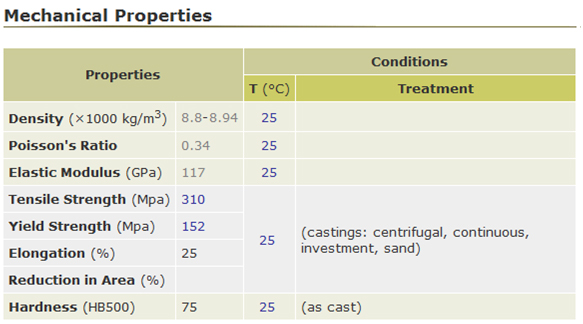Table 6. Tin Bronze properties .

The material information given so far is about the general material design principles of the bending tool especially the pressure die. The given project requires the same material for the each part of the bending tool. Hence it is decided to take the parts as a whole and to consider them all while choosing the material. In compliance with the company visits, the following materials are incorporated into the general comparison.

The comparison of the necessary properties of steels for all bending tools is shown in Table 7.

 Material Hardness rockwell (HRc) Standards Tensile Yield strength (Mpa) Elastic modulus (GPa) Poisson ratio Density (kg/dm3) 1050 Steel 52 (400° F) DIN 1.1210 (AISI 1050) 636 190-210 0.27-0.30 7.7-8.03 2080 Steel 66 DIN 1.2080 (AISI D3) x 194 0,27-0,30 7,70 2379 Steel 62 DIN 1.2379 (AISI D2) 1532 209.9 0,27-0,30 7,67

Table 7. Tool material comparison [9, 10, 17, 18].

As suggested by the companies visited, AISI 1050, AISI D3 and AISI D2 standardized steels are discussed and it is found out that they are the mostly used ones for the bending tool designs. AISI 1050 is chosen out of these three materials with the advantage of being cheaper than the others. As seen in the Table 6 the necessary properties for design such as Elastic Modulus are nearly the same for the three of them. The only difference AISI 1050 steel has is that its tensile yield strength and hardness which are lower than the others. This situation does not lead to a failure of the tool because the strength of the tube is low according to tool

material that does not create critical stresses on tools. Also the material of the tube is ductile which is formable enough to be bent easily. The tool is not in danger of a failure caused by high stresses in bending operations, since the forces applied are very low.

The only problem observed would be the forces that may cause failure of the tube, the calculations are done to prevent this bad result. The only disadvantage about the chosen steel was its hardness which is lower than the others. Generally high hardness is desired in a tool design in order to avoid wears. Wear problems in bending operations are not as crucial as other forming operations as a result of low forces and low chemical affinity so that it is not that necessary to choose the other cold work tool materials since AISI 1050 has a sufficient hardness value.

4.2. Die Calculations

Calculations that are used for tool ion are shown below :

Wall factor: Wall factor is the ratio of bending radius to wall thickness and is used for ing mandrel with the help of "D" of bend ratio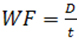(21)

“D” of bend: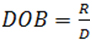(22)

Mandrel nose diameter for single-wall tubing: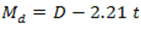(23)ifelse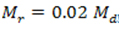(24)(25)

Mandrel ball diameter:(26)

Maximum set-up depth of mandrel nose:Wiper feathered edge thickness:

where it is a constant approaching zero depending upon limitations of material and method of manufacturing.if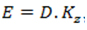elseClamp length: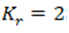where it is a constant for material rigidity..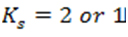where it is a constant limiting the minimum clamp length depending upon the surface of the cavity and the value of 2 for smooth cavities and 1 for serrated cavities.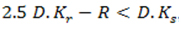ifelse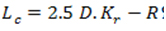(27)

Pressure die length:(28)

Analytical calculations:

In Table 8, results taken from Matlab is shown which was calculated by using die calculations formulas. Wall Factor and “D” of Bend values are used in the further sections for choosing a proper type.

 Definition Value Unit Wall Factor 15 - "D" of Bend 2.33 - Mandrel Diameter 12.79 mm Mandrel Nose Radius 1.28 mm Mandrel Ball Diameter 12.76 mm Max. Set-up Depth 4.23 mm Wiper Edge Thickness 0.04 mm Clamp Length 40 mm Pressure Die Length 84.98 mm

Table 8. Tool calculation results

4.3. Tool ion

4.3.1. Bend die ion

Three types of the bend dies are shown in the Figure 3. The first type is the one with an insert of an interlock. The second type is a bend die that has concentric bore inside and the third type is a one piece die with no inserts.

With respect to the project given the bending machine is a constant parameter of this tool design. One piece bend die is the type suitable with the bending machine out of these three types. More importantly it is shown in the figure that this type has no interlocking part and also no inserted blocks. Furthermore the dies used in the company had non insert interlocking regions and the design is made conforming to the dies currently used and the designs made for the same machine before.

4.3.2. Clamp die ion

The clamp is the part that applies and compensates the forces in a bending tool. The point here is that the clamp should be interlocked with the bend die. There are threads inside of both the clamp die and the bend die that help the die combination to hold the tube powerfully. The holding power is crucial in this operation in order to bend the tube appropriately. Moreover the threads which are located at the both clamp and bend die sides should properly complement each other. On the other hand as shown in the figures because of the bend die type there is no interlocking part but in the given project the combination had non insert interlocking regions. Additionally as shown in the Figure 3 there is a gap between the clamp and the bend die. This gap is generally about 1mm width. The diameter inside is the same with the outer diameter of the tube. That way it is intended to raise the holding force

4.3.3. Pressure die ion

Figure

The pressure die is the part that holds the tube and lets the tube flow while bending operation is happening. The main mission of the pressure die by letting the material flow is to provide the maintainability of the tube?s dimensions without losing its shape.

4.3.4. Mandrel ion

For mandrel ion “D” of bend and wall factor is important. The “D” of bend is calculated as 2.33 (taken as 2.5) and the wall factor is calculated as 15 that can be seen in Die calculations section. According to these values the appropriate mandrel is a plug mandrel as shown in the ion chart (Table 9).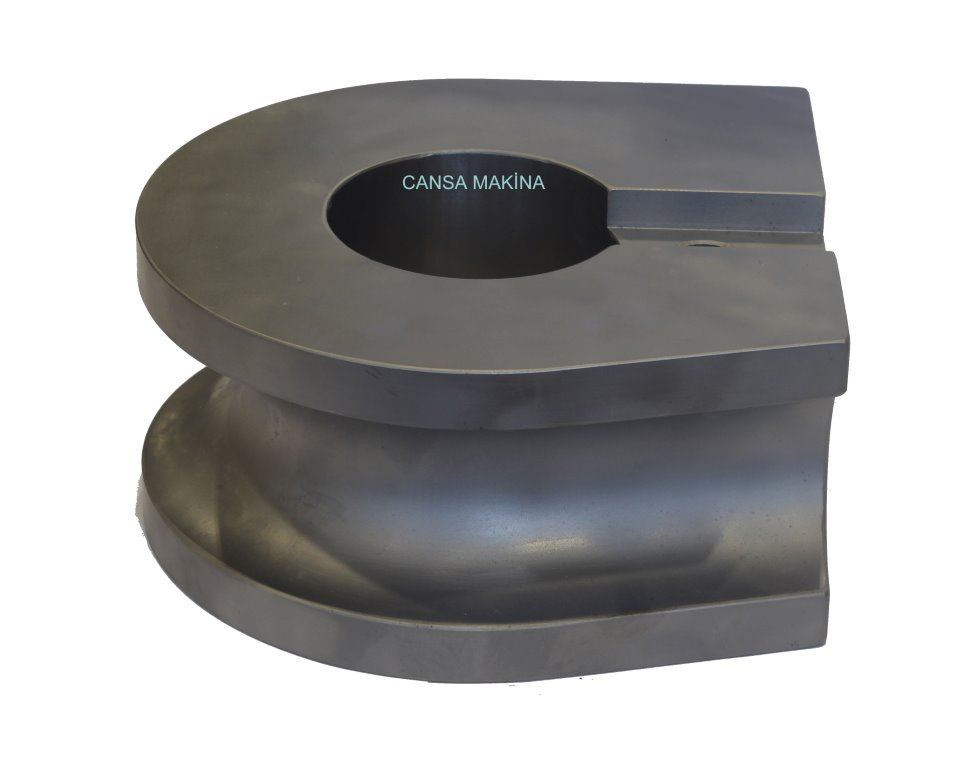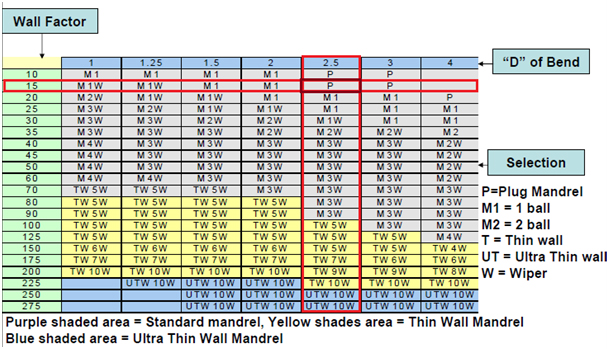Table 9. Mandrel ion chart .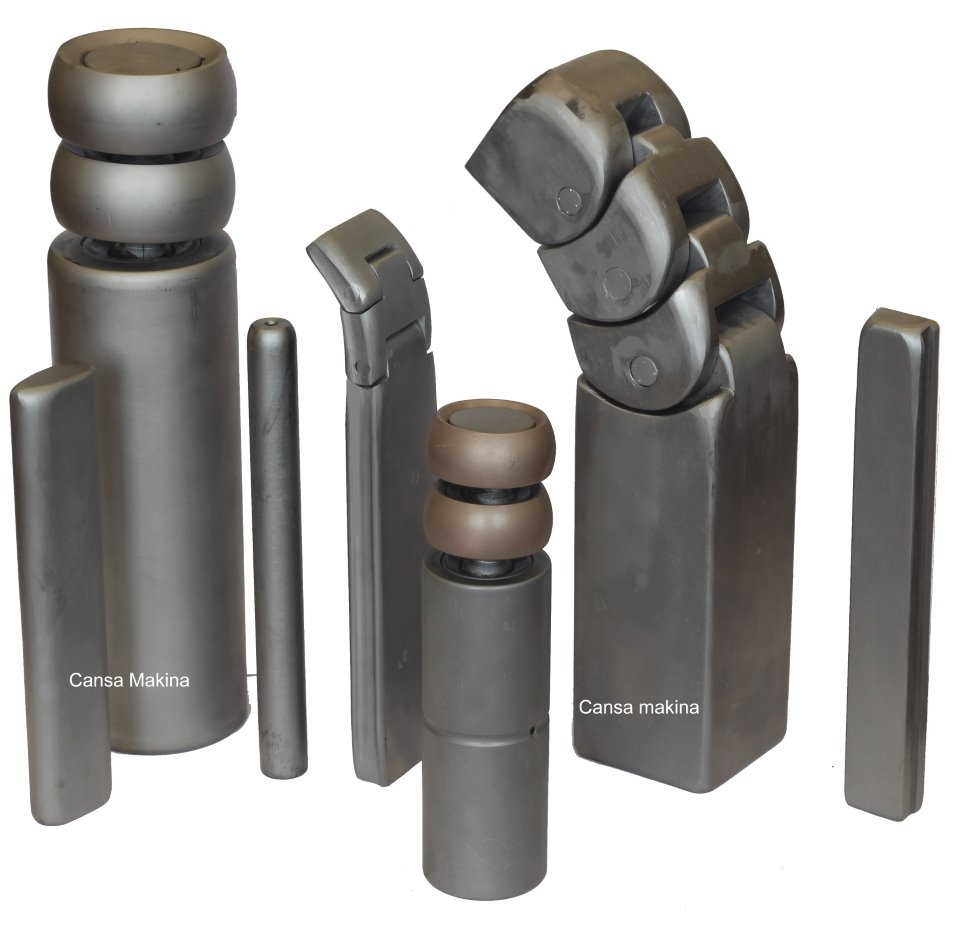Figure 23. Mandrel types .

Mandrels with balls provides better geometries while bending especially for the tubes of which diameter to thickness ratios are too large. Disadvantage of these ball mandrels is that they have less tool life than plug mandrels.

Technical drawings for manufacturing are given in Appendix A.

1. TubeFigür 24. Boru

2. Die bendFigür 25. bend die

3. clamp dieFigür 26. clamp die

4. Pressure DieFigür 27. Pressure Die

5. MandrelFigür 28. Ball Mandrel

6.Bending SystemFigür 29. Bending System.

III. ANALYSIS

1.     Overbend Calculator

Overbend Calculator is a program for finding the necessary bend angle considering springback. First step is Bender Setup, where you enter the degree of bending (Figure30). This value is currently used by company and acquired from previous bending processes. For example to get a 90 degree bended tube they bend 92.6 degrees. Second Step is Measure Bends, where you enter the degree after bending when springback occurs (Figure 31). In third step the program generates formula values (Figure32) and at the final step you can see the results. The Overbend page gives you the angle of bend you must enter to the machine according to desired bend angle (Figure 33).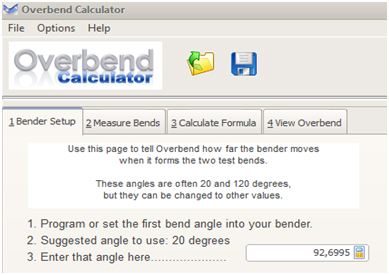Figure 30. First step.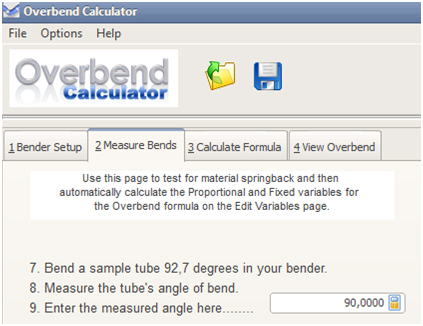Figure 31. Second step.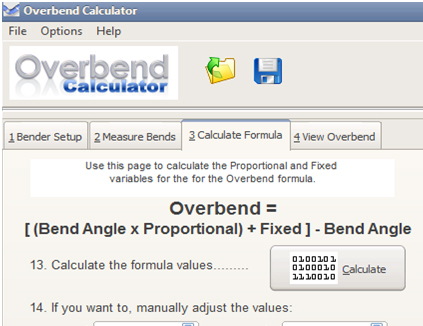Figure 32. Formula values.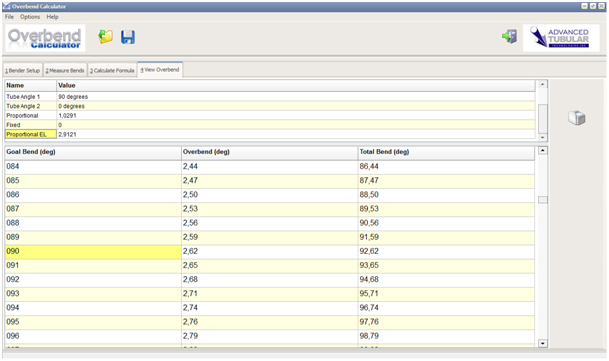Figure 33. Overbend Angle.

2. Dynaform

eta/DYNAFORM Version 5.7.3 software is an LS-DYNA-based sheet metal forming simulation solution package developed by Engineering Technology Associates, Inc. Dynaform has a Quick Setup module as a Rotary Draw Bending shown in Figure 34. After drawing each of the tool and tube as shell by using Surface Tools on SolidWorks and saving them in the .IGS format, every part is imported into Dyanaform one by one. Tools and tube material are meshed as shown in Figure 34 and defined separately in the Rotary Draw Bending Module. This module assumes tooling materials as rigid and also contact interface between tools and tube are „forming_surface_to_surface?. After analyses finished, the results is examined with the Post-Processor for Daynaform as shown in Figure 35. This finit element analysis results are discussed in the further sections.Figure 34. Dynaform Rotary Draw Bending Quick Setup.Figure 35. Dynaform Post-Process.

4.1. FLD (Forming Limit Diagram)

Forming Limit Diagram shows the behavior of the material to predict the formation of cracks and wrinkles. The mathematical expression is: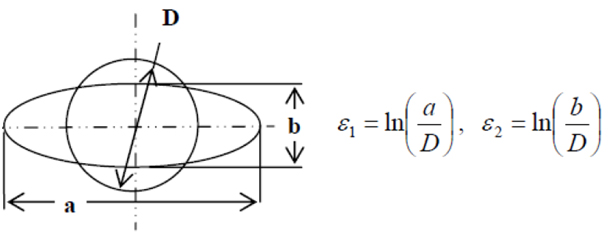Figure 36. Definition of major and minor strain .

As shown in Figure 36, bigger value ε1 is considered as the major strain and placed in vertical axis. Smaller value ε2 is considered as the minor strain and placed in the horizontal axis in the Forming Limit Diagram.Figure 37. Forming Limit Diagram.Figure 38. Change in the geometry around bending area.

As it was mentioned in the previous sections due to the compressive stresses in the inner section of the tube walls, wrinkle formation tendency can be seen, as in Figure 37 and Figure 38 colored pink and purple. Also the cross-section of the tube changes while bending. The shape of the tube in the bending area is not an exact circle anymore. The change in the geometry can be seen clearly in Figure 38. From the Forming Limit Diagram, it is observed that the bending operation is very far away from the risk of crack. Therefore this bending process is safe under these conditions as desired and calculated before.

4.2. von Mises Criteria

von Mises criteria combines the three principle stresses to compare with yield strength of the material. The von Mises stress satisfies the property that two stress states with equal distortion energy have equal von Mises stress. The mathematical expression is:(29)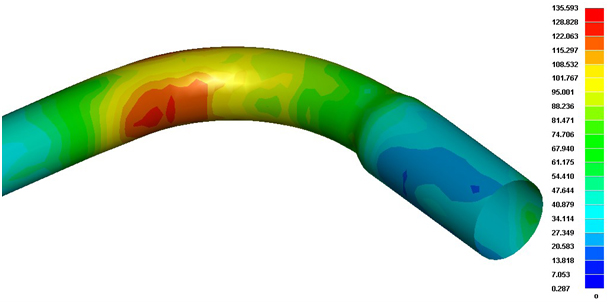Figure 39. – 40. Von Mises Stress distribution.

After bending operation, since tube bending is a plastic deformation process, stresses shown in Figure 39 and Figure 40 are the residual stresses. These stresses are small compared to yield strength of brass.

4.3. Thickness

Thickness distribution is one of the major concerns in the bended area that affects the strength of the tube directly. Therefore this distribution should be examined carefully.Figure 41. Thickness distribution on the outer semi-circle.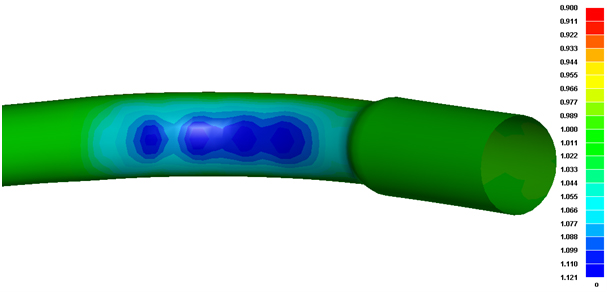Figure 42. Thickness distribution on the inner semi-circle.

As mentioned before, due to tensile strength in the outer section, wall thickness of the tube decreases from 1mm to 0.9 mm the same as found in the analytical calculations. Thickness distribution can be seen and read from the scale in Figure 41. Also because of the compressive stress in the inner section, maximum wall thickness increases up to 1.12 mm shown in Figure 42, that is very close to the analytical solution of 1.16 mm.

4.4. Edge MovementFigure 43. Edge Movement.

Figure 43 shows the edge movement of the tube according to the starting position. In other words, for the edge on the left of the Figure 43 means preparation length that was calculated as 56.51 mm in the analytical calculations before and also closely fits with the results as navy blue in Figure 43.

4.5. Forces

3.5. KuvvetlerFigure 44. Forces on tools.

Forces acting on tools are plotted in Figure 44. It can be seen that values on X and Y axis are not critical to deform tool material. Due to the bending geometry there is no force acting on tools on Z direction. Since there is no chance to give degrees of freedom for mandrel while setting up the model in Dynaform, all forces acting on mandrel in the graph will act on pressure die in reality. This forces show the importance of wear resistance rather than tool strength especially at high amount of bending operations.

Prepared

Ceren ÇELEBİ

Merve DEDE

Serdar SEÇKİN

Elif HAMLACIOĞLU

PARTICIPANT COMPANY

Cansa Makina San. Ve Tic. Ltd. Şti. – Boru bükme Makinası Üreticisi

Mersedes-Benz Türk – Boru Bükme makinası Kullanıcısı